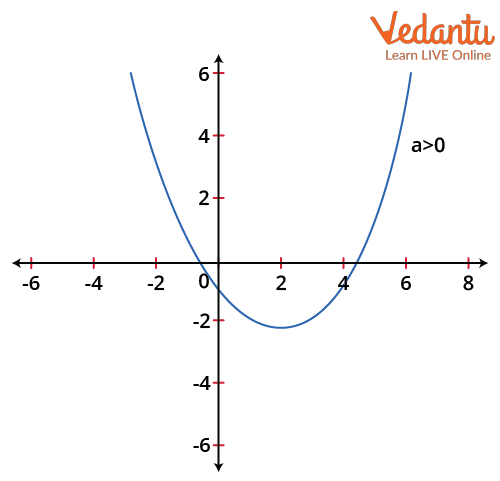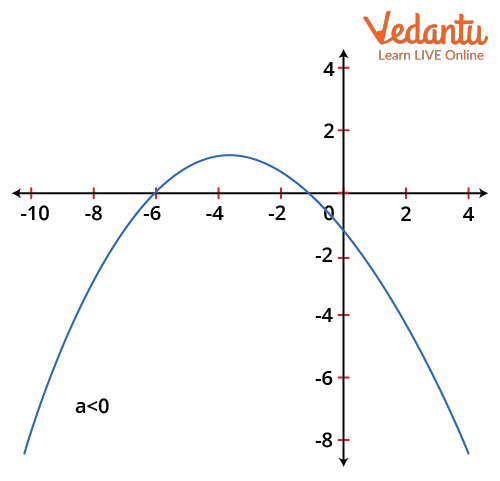Courses
Courses for Kids
Free study material
Free LIVE classes
More

# Maximum and Minimum Value of a Quadratic Polynomial With Solved Examples for JEELIVE
Join Vedantu’s FREE Mastercalss

Quadratic equations are a crucial part of the IIT JEE Mathematics curriculum. Quadratic Polynomial is the Polynomial in which the highest power of the variable is 2 with the condition that the coefficient of the variable with the power of 2 must not be zero.

The general equation of a Quadratic polynomial is:

$a x^{2}+b x+c=0$

Therefore, in this equation, a can be negative or positive but must not be zero; however, b and c can take any value.

### Solution of a Quadratic Polynomial

The formula for finding the roots or solution of a quadratic equation is derived by the Indian mathematician Sridharachya (c.750 CE – c.930CE) is:

$x=\dfrac{-b \pm \sqrt{b^{2}-4 a c}}{2 a}$

So, the two roots of the quadratic equation are:

$x=\dfrac{-b + \sqrt{b^{2}-4 a c}}{2 a}$ and $x=\dfrac{-b - \sqrt{b^{2}-4 a c}}{2 a}$

Proof of the formula:

Since the given Quadratic equation is,

$a x^{2}+b x+c=0$

Divide the equation by a,

$x^{2}+\dfrac{b}{a} x+\dfrac{c}{a}=0$

Now, solve the expression by completing the square method,

$x^{2}+2 \times \dfrac{b}{2 a} x=-\dfrac{c}{a} \\ \Rightarrow x^{2}+2 \times \dfrac{b}{2 a} x+\left(\dfrac{b}{2 a}\right)^{2}-\left(\dfrac{b}{2 a}\right)^{2}=-\dfrac{c}{a} \\ \Rightarrow \left(x^{2}+2 \times \dfrac{b}{2 a} x+\left(\dfrac{b}{2 a}\right)^{2}\right)=\left(\dfrac{b}{2 a}\right)^{2}-\dfrac{c}{a} \\ \Rightarrow \left(x-\dfrac{b}{2 a}\right)^{2}=\dfrac{b^{2}-4 a c}{4 a^{2}} \\ \Rightarrow x-\dfrac{b}{2 a}=\pm \sqrt{\dfrac{b^{2}-4 a c}{4 a^{2}}} \\ x=\dfrac{-b \pm \sqrt{b^{2}-4 a c}}{2 a}$

Since the value of any variable under the root can not be negative, therefore, in the expression $\sqrt{b^{2}-4 a c}$, $b^{2}-4 a c \geq 0$,it is called the discriminant.

Discriminant tells the type of the roots for a given quadratic equation,

1. For $b^{2}-4 a c > 0$, there will be two distinct roots of the equation.

2. For $b^{2}-4 a c = 0$, there will be two equal roots.

3. For $b^{2}-4 a c < 0$, there will be no real root existing for the equation but

there will be two imaginary(complex) roots.

### Maximum Value of Quadratic Equation

There are two cases of finding the maximum value of a quadratic equation.

1. For a > 0

For a > 0, the graph of the quadratic equation will be open upwards as shown in the image below.Graph of the quadratic equation for a > o

From the graph, the maximum value is not defined as increasing the value of x the graph approaches infinity.

2. For a < 0

For a < 0, the graph of the quadratic equation will open downwards as shown in the image below.Graph of the quadratic equation for a<o

To find the maximum value, let $y=a x^{2}+b x+c$,

$\Rightarrow a x^{2}+b x+c-y=0$

Since the solution of a quadratic equation is,

$x=\dfrac{-b \pm \sqrt{b^{2}-4 a c}}{2 a}$

Substitute c to c-y in the equation,

$x=\dfrac{-b \pm \sqrt{b^{2}-4 a(c-y)}}{2 a}$

Since the discriminant must be positive,

$D=b^{2}-4 a(c-y) \geq 0 \\ \Rightarrow b^{2}-4 a c+4 a y \geq 0 \\ \Rightarrow 4 a y \geq 4 a c-b^{2}$

Since a < 0 so multiply (-1) on both sides of the inequality to make the expression positive.

$-4 a y \leq b^{2}-4 a c \\ \Rightarrow y \leq \dfrac{b^{2}-4 a c}{-4 a} \\ \Rightarrow y \leq \dfrac{-b^{2}}{4 a}+c \\ \Rightarrow y \leq c-\dfrac{b^{2}}{4 a}$

(Note: on dividing -4a both sides of the inequality, the sign of the inequality doesn’t change because -4a > 0, as a < 0.)

So, the maximum value attained is $y=c-\dfrac{b^{2}}{4 a}$

Now find the value of x to the corresponding maximum value of y

$x=\dfrac{-b \pm \sqrt{b^{2}-4 a(c-y)}}{2 a}\\ \Rightarrow x=\dfrac{-b \pm \sqrt{b^{2}-4 a c+4 a\left(c-\dfrac{b^{2}}{4 a}\right)}}{2 a}\\ \Rightarrow x=\dfrac{-b \pm \sqrt{b^{2}-4 a c+4 a c-b^{2}}}{2 a}\\ x=\dfrac{-b}{2 a}$

So, For a < 0 the quadratic equation will attain its maximum value at,

$x=\dfrac{-b}{2a}$

## Minimum Value of Quadratic Equation

Similarly, there are two cases of finding the minimum value of a quadratic equation.

1. For a > 0

From the graph of the quadratic polynomial for a > 0, there will be a finite value for which the graph attains its minimum value.

To find the minimum value, let $y=a x^{2}+b x+c$

$\Rightarrow a x^{2}+b x+c-y=0$

Since the discriminant of the quadratic equation must be positive,

$D=b^{2}-4 a(c-y) \geq 0 \\ \Rightarrow b^{2}-4 a c+4 a y \geq 0 \\ \Rightarrow 4 a y \geq 4 a c-b^{2} \\ \Rightarrow y \geq \dfrac{4 a c-b^{2}}{4 a} \\ \Rightarrow y \geq c-\dfrac{b^{2}}{4 a}$

Therefore the minimum value attained by y is $y=c-\dfrac{b^{2}}{4 a}$.

Now find the value of x to the corresponding maximum value of y,

$x=\dfrac{-b \pm \sqrt{b^{2}-4 a(c-y)}}{2 a}\\ \Rightarrow x=\dfrac{-b \pm \sqrt{b^{2}-4 a c+4 a\left(c-\dfrac{b^{2}}{4 a}\right)}}{2 a}\\ \Rightarrow x=\dfrac{-b \pm \sqrt{b^{2}-4 a c+4 a c-b^{2}}}{2 a}\\ x=\dfrac{-b}{2 a}$

So, For a > 0, the quadratic equation will attain its maximum value at,

$x=\dfrac{-b}{2 a}$

2. For a < 0

From the graph of the quadratic polynomial a > 0, there will be no finite value x for which the graph attains its minimum value, since increasing the value of x the graph approaches the negative of infinity.

## Solved Examples

Example 1: Determine the minimum and maximum values of the quadratic function below.

$f(x)=2 x^{2}+7 x+5$

Solution:

The parabola is open upward because the x2 coefficient is positive. As a result, the function will only have one value, which is the vertex y-coordinate.

To determine the vertex y-coordinate, we must first determine the vertex's x-coordinate.

The formula for determining the vertex's x-coordinate is $=\dfrac{-b}{2 a}$

Given us, a = 2, b = 7

Then, The formula for determining the vertex's x-coordinate is

$=\dfrac{-7}{2 \times 2} \\ \Rightarrow\dfrac{-7}{4}$

Substitute $\dfrac{-7}{4}$ for x in the above function to get the vertex y-coordinate, the y-coordinate;

$\Rightarrow f\left(\dfrac{-7}{4}\right) \\ \Rightarrow 2\left(\dfrac{-7}{4}\right)^{2} + 7\left(\dfrac{-7}{4}\right) + 5 \\ \Rightarrow 2\left(\dfrac{49}{16}\right) - \dfrac{49}{4} + 5 \\ \Rightarrow \dfrac{98}{16} - \dfrac{49}{4} + 5 \\ \Rightarrow \dfrac{-9}{8}$

Ans: $\dfrac{-9}{8}$ is the minimal value.

Example 2: Determine the minimum and maximum values of the quadratic function below.

$f(x)=-5 x^{2}+30 x+200$

Solution:

The parabola is open downward because the x2 coefficient is negative. As a result, the function will only have one maximum value, which is the vertex y-coordinate.

To determine the vertex y-coordinate, we must first determine the vertex's x-coordinate. The formula for determining the vertex's x-coordinate is $=\dfrac{-b}{2a}$

Given us, a= -5, b= 30

The formula for determining the vertex's x-coordinate is

$\Rightarrow \dfrac{-(-30)}{2 \times 5} \\ \Rightarrow \dfrac{30}{10} =3$

Substitute 3 for x in the above function to get the vertex y-coordinate.

The y-coordinate,

$\Rightarrow f(3) \\ \Rightarrow -5\left(3^{2}\right)+30(3)+200 \\ \Rightarrow -5(3 \times 3)+90+200 \\ \Rightarrow -5(9)+290 \\ \Rightarrow -45+290 =245$

Ans: = 245 is the maximum value.

## Conclusion

A quadratic polynomial is a polynomial in which the highest power of the variable is 2 and adding the condition that the coefficient of the variable with power 2 must not be zero. The solution of a quadratic polynomial is $x=\dfrac{-b \pm \sqrt{b^{2}-4 a c}}{2 a}$, $b^2-4ac$ is called discriminant and it must be positive to get a real root of x.

From the two cases,

1. For a > 0, the maximum and minimum value of quadratic equation for this case is that the maximum value is not defined as increasing the value of x, it approaches infinity and the minimum value is attained at $x=\dfrac{-b}{2 a}$.

2. For a < 0, the maximum and the minimum value of quadratic equation for this case is that the minimum value is not defined as increasing the value of x, it approaches negative of infinity and the maximum value is attained at $x=\dfrac{-b}{2 a}$.

Last updated date: 17th Sep 2023
Total views: 133.2k
Views today: 3.33k

## FAQs on Maximum and Minimum Value of a Quadratic Polynomial With Solved Examples for JEE

1. How can we define a quadratic equation?

A second-degree equation of the form $ax^2+bx+c=0$ is known as a quadratic equation in mathematics. Here, x is the variable, c is the constant term, and a and b are the coefficients. There are two roots or solutions to this quadratic equation because the variable x is of the second degree. Either factoring the solution or applying the quadratic formula will reveal the quadratic equation's roots.  The formula to find the solution of a Quadratic equation is:

$x=\dfrac{-b \pm \sqrt{b^{2}-4 a c}}{2 a}$

2. How to do a step-by-step solution for a quadratic equation?

Factoring, using square roots, completing the square, and the quadratic formula are the four ways to solve a quadratic problem.

•  $ax^2+bx+c=0$ is the quadratic equation in standard form. Find the values of a, b, and c.

• The quadratic formula in writing. Then enter the values for a, b, and c.

• You can factor quadratic equations.

•  The formula for a quadratic: $x=\dfrac{-b \pm \sqrt{b^{2}-4 a c}}{2 a}$

• There are two viable solutions when the discriminant ($b^2-4ac$) is positive. There is only one effective remedy, zero. negative, there are two difficult fixes.